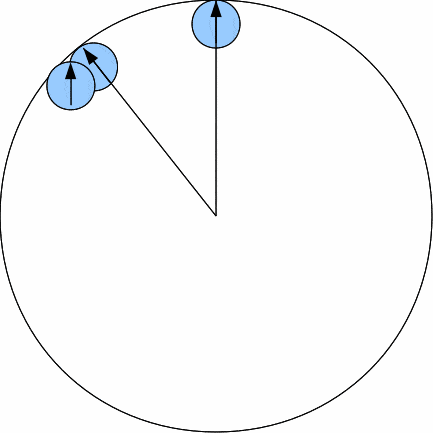# Rotating tangent circles

• sparkle123
In summary, the smaller circle needs to make 7 rotations around the larger circle before it is back in the same position relative to it. If the smaller circle is traveling on the outside of the larger circle, it will need 9 rotations.f

#### sparkle123

I've been thinking about these problems for a long time but I really can't wrap my mind around them. Please share your insights!

Consider a circle of radius R/8 internally tangent (inside) a circle of radius R. How many rotations does it take for small circle to return to the same position? Does it matter whether or not the big circle is fixed in place? I got that only 7 revolutions are required from a solution to a problem that is slightly different.

What about if the smaller circle was traveling on the outside of the larger circle, so that it's externally tangent? Again, does it matter whether or not the big circle is fixed in place?

Thank you!

The number of rotations is equal to the ratio of the radii of the two circles, it doesn't matter if the large circle is moving so long as the smaller circle moves with it.
To see why consider the small circle, each time it rotates it covers a distance $2 \pi \frac{R}{8}$, for it to make it all the way around the larger circle it must cover a distance equal to the circumference of the larger circle $2 \pi R$. So the number of rotations is just the larger circumference divided by the smaller one, which simplifies to the larger radius divided by the smaller.
As a bit of a brain teaser consider the opposite scenario where the larger circle rotates, staying tangent to the smaller one, how many rotations will it need for the smaller one to be back in the same place relative to the larger one? :)

Last edited:
Hi sparkle123!The number of revolutions relative to the large circle is 8, which is the ratio of the circumferences, which in turn is the ratio of the radii.
The same holds whether it is inside or outside.

However, if you look at absolute revolutions, it is 7 for inside, and 9 for outside.

This is similar to the Earth making its revolutions.
It matters whether you count the days, or the actual full revolutions.
The Earth makes 366.25 full revolutions in a year, but you only have 365.25 days in a year.
The so called "sidereal day" is 23 hours, 56 minutes, and 4.1 seconds.
This is the time it takes the Earth to make a revolution "as observed from the side", instead of "as observed relative to the sun".

Thanks JHamm and I like Serena, and hi I like Serena! :)

@I like Serena: Does this mean that the absolute number of rotations is always one less than the number of rotations relative to the large circle? What if the larger circle's radius is not a whole number multiple of the smaller circle's?
Also, do you know a good way of "seeing this"? Thanks so much!

Thanks JHamm and I like Serena, and hi I like Serena! :)

@I like Serena: Does this mean that the absolute number of rotations is always one less than the number of rotations relative to the large circle? What if the larger circle's radius is not a whole number multiple of the smaller circle's?
Also, do you know a good way of "seeing this"? Thanks so much!

In your case it's always exactly +1 or -1.

To "see" this, you need to make a proper drawing!

Here's a picture showing how you can "see" it.When you "roll" one revolution of the small circle along the large circle, it is aligned again with the radius of the large circle.
You have to roll it slightly further to make a full revolution.

When you go full circle, you will be exactly 1 revolution short, counted in absolute revolutions.

#### Attachments

I totally got it! Thank you! :)